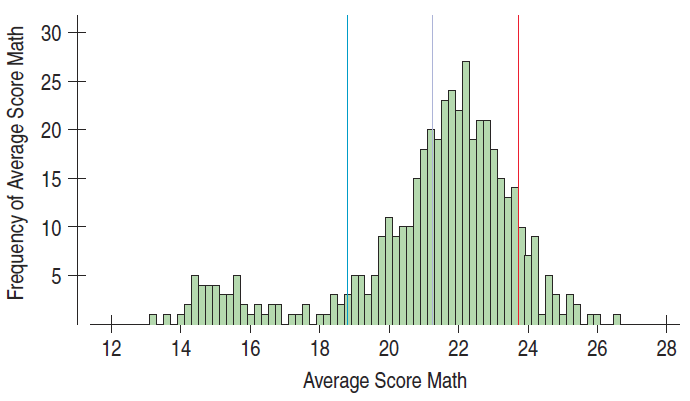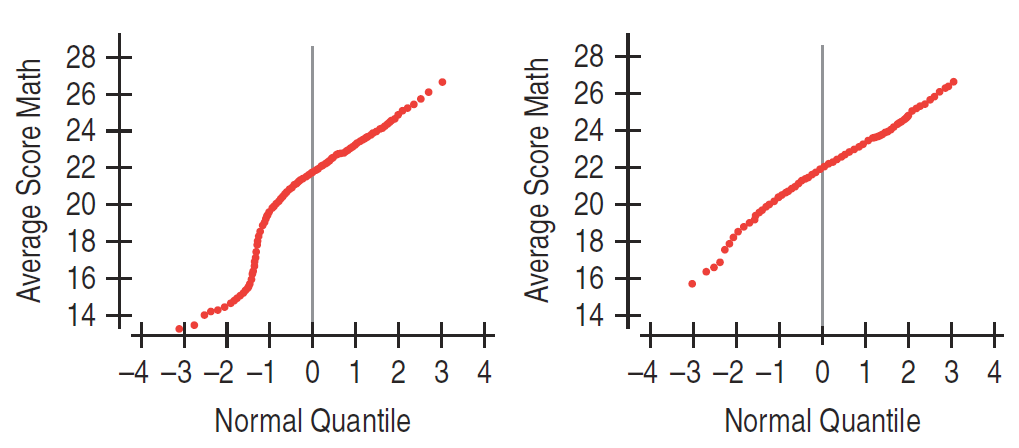×
Get Full Access to Stats: Data And Models - 4 Edition - Chapter 5 - Problem 12e
Get Full Access to Stats: Data And Models - 4 Edition - Chapter 5 - Problem 12e

×

# Wisconsin ACT math The histogram shows the distribution ofISBN: 9780321986498 70

## Solution for problem 12E Chapter 5

Stats: Data and Models | 4th Edition

• Textbook Solutions
• 2901 Step-by-step solutions solved by professors and subject experts
• Get 24/7 help from StudySoup virtual teaching assistantsStats: Data and Models | 4th Edition

4 5 1 283 Reviews
10
1
Problem 12E

Problem 12E

Wisconsin ACT math The histogram shows the distribution of mean ACT mathematics scores for all Wisconsin public schools in 2011. The vertical lines show the mean and one standard deviation above and below the mean. 78.8% of the data points are between the two outer lines.

a) Give two reasons that a Normal model is not appropriate for these data.b) The Normal probability plot on the left shows the distribution of these scores. The plot on the right shows the same data with the Milwaukee area schools (mostly in the low mode) removed. What do these plots tell you about the shape of the distributions?Step-by-Step Solution:

Problem 12E

Wisconsin ACT math The histogram shows the distribution of mean ACT mathematics scores for all Wisconsin public schools in 2011. The vertical lines show the mean and one standard deviation above and below the mean. 78.8% of the data points are between the two outer lines.

a) Give two reasons that a Normal model is not appropriate for these data.b) The Normal probability plot on the left shows the distribution of these scores. The plot on the right shows the same data with the Milwaukee area schools (mostly in the low mode) removed. What do these plots tell you about the shape of the distributions?Step by Step Solution

Step 1 of 2

(a)

According to the 68-98-99.7 rule. In a Normal model, about 68% of the values fall within 1 standard deviation of the mean. But here it’s given that 78.8% fall within 1 standard deviation of the mean. Therefore, the Normal model is not appropriate for these data.

The distribution is left skewed because the highest bar is on the right side of the histogram. Normal models are appropriate for distributions whose shapes are unimodal and roughly symmetric. So Normal model is not appropriate for these data

Step 2 of 2

##### ISBN: 9780321986498

Stats: Data and Models was written by and is associated to the ISBN: 9780321986498. Since the solution to 12E from 5 chapter was answered, more than 495 students have viewed the full step-by-step answer. This full solution covers the following key subjects: mean, these, shows, data, Schools. This expansive textbook survival guide covers 12 chapters, and 679 solutions. The full step-by-step solution to problem: 12E from chapter: 5 was answered by , our top Statistics solution expert on 08/30/17, 07:04AM. This textbook survival guide was created for the textbook: Stats: Data and Models , edition: 4. The answer to “Wisconsin ACT math The histogram shows the distribution of mean ACT mathematics scores for all Wisconsin public schools in 2011. The vertical lines show the mean and one standard deviation above and below the mean. 78.8% of the data points are between the two outer lines.a) Give two reasons that a Normal model is not appropriate for these data. b) The Normal probability plot on the left shows the distribution of these scores. The plot on the right shows the same data with the Milwaukee area schools (mostly in the low mode) removed. What do these plots tell you about the shape of the distributions?” is broken down into a number of easy to follow steps, and 105 words.

Unlock Textbook Solution